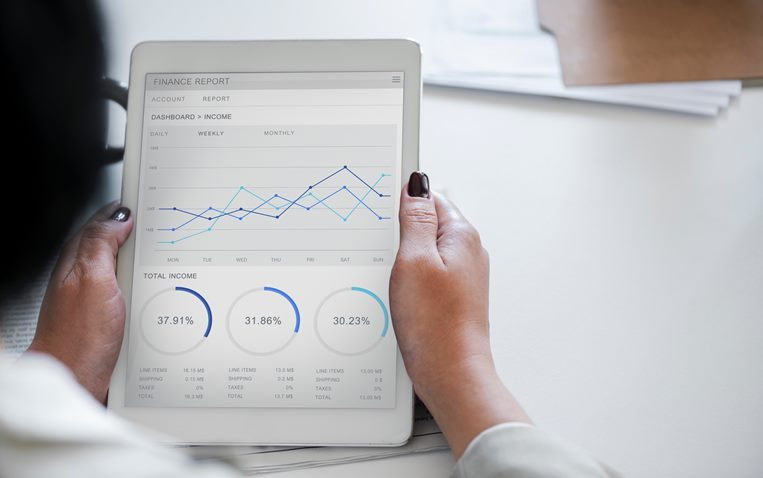# How to calculate rental yield

Rental yield is a simple calculation to determine the return on investment that a property can produce. Some investors are willing to purchase a property sight unseen based on rental yield (as crazy as that may seem).

However, rental yields alone should not be used as an indicator for a sound investment property as there are many variables which can impact yields. Looking at statistics in isolation may hide vital facts and trends that need to be taken into consideration for a sound investment.

Additionally, rental yields will tend to be lower in a higher value property so understanding your strategy is more important than just looking at one element of the transaction.

There are two rental yield calculation you can look at, these are net rental yield and gross rental yield.

Gross rental yield is the more common calculation used to determine the return on investment for residential property and is commonly the yield which is promoted as part of the value of an investment purchase. You may see this promoted when speaking with a buyers agent or a sales agent in their marketing material.

To calculate the gross rental yield, you need to take the total income from the property and divide that by the value or the property then multiply this number by 100.

For example:

• Total income from rent = \$22,000
• Property value is \$500,000
• Calculation is \$22,000 divided by \$500,000 = 0.044 x 100 = 4.4%

A gross rental yield of 8% is generally the magic number to have cash going back into your pocket. However depending on the property, the loan amount, repayments etc, you can have a positive cash flow property at lower yields.

Net rental yield is the calculation of net income divided by the properties purchase price and is commonly used for commercial property as the tenant pays for all expenses (not the owner). To calculate these numbers you need to deduct all the expenses, such as management fees, rates and costs to maintain the property from the annual rental income (i.e. total income less total expenses). You then divide that number by the property's purchase price and times it by 100. This will give you the rental yield as a percentage.